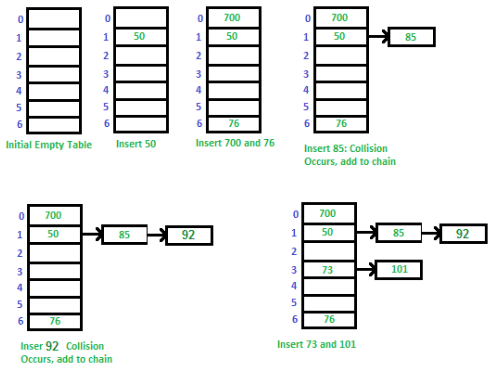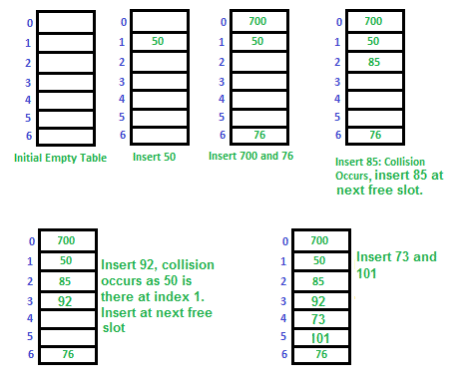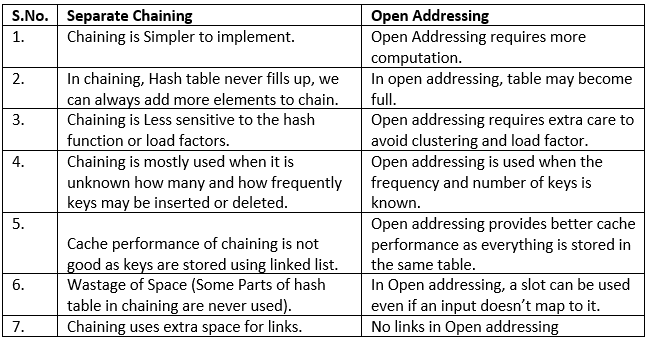# Hashing Notes | Study Algorithms - Computer Science Engineering (CSE)

## Computer Science Engineering (CSE): Hashing Notes | Study Algorithms - Computer Science Engineering (CSE)

The document Hashing Notes | Study Algorithms - Computer Science Engineering (CSE) is a part of the Computer Science Engineering (CSE) Course Algorithms.
All you need of Computer Science Engineering (CSE) at this link: Computer Science Engineering (CSE)

Introduction

Suppose we want to design a system for storing employee records keyed using phone numbers. And we want following queries to be performed efficiently:

1. Insert a phone number and corresponding information.
2. Search a phone number and fetch the information.
3. Delete a phone number and related information.

We can think of using the following data structures to maintain information about different phone numbers.

1. Array of phone numbers and records.
2. Linked List of phone numbers and records.
3. Balanced binary search tree with phone numbers as keys.
4. Direct Access Table.

For arrays and linked lists, we need to search in a linear fashion, which can be costly in practice. If we use arrays and keep the data sorted, then a phone number can be searched in O(Logn) time using Binary Search, but insert and delete operations become costly as we have to maintain sorted order.
With balanced binary search tree, we get moderate search, insert and delete times. All of these operations can be guaranteed to be in O(Logn) time.
Another solution that one can think of is to use a direct access table where we make a big array and use phone numbers as index in the array. An entry in array is NIL if phone number is not present, else the array entry stores pointer to records corresponding to phone number. Time complexity wise this solution is the best among all, we can do all operations in O(1) time. For example to insert a phone number, we create a record with details of given phone number, use phone number as index and store the pointer to the created record in table.
This solution has many practical limitations. First problem with this solution is extra space required is huge. For example if phone number is n digits, we need O(m * 10n) space for table where m is size of a pointer to record. Another problem is an integer in a programming language may not store n digits.
Due to above limitations Direct Access Table cannot always be used. Hashing is the solution that can be used in almost all such situations and performs extremely well compared to above data structures like Array, Linked List, Balanced BST in practice. With hashing we get O(1) search time on average (under reasonable assumptions) and O(n) in worst case.
Hashing is an improvement over Direct Access Table. The idea is to use hash function that converts a given phone number or any other key to a smaller number and uses the small number as index in a table called hash table.

Hash Function: A function that converts a given big phone number to a small practical integer value. The mapped integer value is used as an index in hash table. In simple terms, a hash function maps a big number or string to a small integer that can be used as index in hash table.

A good hash function should have following properties:

1. Efficiently computable.
2. Should uniformly distribute the keys (Each table position equally likely for each key)

For example for phone numbers a bad hash function is to take first three digits. A better function is consider last three digits. Please note that this may not be the best hash function. There may be better ways.

Hash Table: An array that stores pointers to records corresponding to a given phone number. An entry in hash table is NIL if no existing phone number has hash function value equal to the index for the entry.

Collision Handling: Since a hash function gets us a small number for a big key, there is possibility that two keys result in same value. The situation where a newly inserted key maps to an already occupied slot in hash table is called collision and must be handled using some collision handling technique. Following are the ways to handle collisions:

• Chaining: The idea is to make each cell of hash table point to a linked list of records that have same hash function value. Chaining is simple, but requires additional memory outside the table.
• Open Addressing: In open addressing, all elements are stored in the hash table itself. Each table entry contains either a record or NIL. When searching for an element, we one by one examine table slots until the desired element is found or it is clear that the element is not in the table.

Separate Chaining

What is Collision?
Since a hash function gets us a small number for a key which is a big integer or string, there is a possibility that two keys result in the same value. The situation where a newly inserted key maps to an already occupied slot in the hash table is called collision and must be handled using some collision handling technique.

What are the chances of collisions with large table?
Collisions are very likely even if we have big table to store keys. An important observation is Birthday Paradox. With only 23 persons, the probability that two people have the same birthday is 50%.

How to handle Collisions?
There are mainly two methods to handle collision:

• Separate Chaining

1. Separate Chaining: The idea is to make each cell of hash table point to a linked list of records that have same hash function value.
Let us consider a simple hash function as “key mod 7” and sequence of keys as 50, 700, 76, 85, 92, 73, 101.C++ program for hashing with chaining

• Simple to implement.
• Hash table never fills up, we can always add more elements to the chain.
• Less sensitive to the hash function or load factors.
• It is mostly used when it is unknown how many and how frequently keys may be inserted or deleted.

• Cache performance of chaining is not good as keys are stored using a linked list. Open addressing provides better cache performance as everything is stored in the same table.
• Wastage of Space (Some Parts of hash table are never used)
• If the chain becomes long, then search time can become O(n) in the worst case.
• Uses extra space for links.

Performance of Chaining: Performance of hashing can be evaluated under the assumption that each key is equally likely to be hashed to any slot of table (simple uniform hashing).
m = Number of slots in hash table
n = Number of keys to be inserted in hash table
Expected time to search = O(1 + α)
Expected time to delete = O(1 + α)
Time to insert = O(1)
Time complexity of search insert and delete is
O(1) if  α is O(1)

Data Structures For Storing Chains:

• Search: O(l) where l = length of linked list
• Delete: O(l)
• Insert: O(l)
• Not cache friendly
• Dynamic Sized Arrays ( Vectors in C++, ArrayList in Java, list in Python)
• Search: O(l) where l = length of array
• Delete: O(l)
• Insert: O(l)
• Cache friendly
• Self Balancing BST ( AVL Trees, Red Black Trees)
• Search: O(log(l))
• Delete: O(log(l))
• Insert: O(l)
• Not cache friendly

2. Open Addressing: Like separate chaining, open addressing is a method for handling collisions. In Open Addressing, all elements are stored in the hash table itself. So at any point, the size of the table must be greater than or equal to the total number of keys (Note that we can increase table size by copying old data if needed).
Insert(k): Keep probing until an empty slot is found. Once an empty slot is found, insert k.
Search(k): Keep probing until slot’s key doesn’t become equal to k or an empty slot is reached.
Delete(k): Delete operation is interesting. If we simply delete a key, then the search may fail. So slots of deleted keys are marked specially as “deleted”.
The insert can insert an item in a deleted slot, but the search doesn’t stop at a deleted slot.
Open Addressing is done in the following ways:
(i) Linear Probing: In linear probing, we linearly probe for next slot. For example, the typical gap between two probes is 1 as seen in the example below.
Let hash(x) be the slot index computed using a hash function and S be the table size
If slot hash(x) % S is full, then we try (hash(x) + 1) % S
If (hash(x) + 1) % S is also full, then we try (hash(x) + 2) % S
If (hash(x) + 2) % S is also full, then we try (hash(x) + 3) % S
..................................................
..................................................
Let us consider a simple hash function as “key mod 7” and a sequence of keys as 50, 700, 76, 85, 92, 73, 101.Challenges in Linear Probing:

• Primary Clustering: One of the problems with linear probing is Primary clustering, many consecutive elements form groups and it starts taking time to find a free slot or to search for an element.
• Secondary Clustering: Secondary clustering is less severe, two records only have the same collision chain (Probe Sequence) if their initial position is the same.

(ii) Quadratic Probing We look for i2‘th slot in i’th iteration.
Let hash(x) be the slot index computed using hash function.
If slot hash(x) % S is full, then we try (hash(x) + 1 * 1) % S
If (hash(x) + 1 * 1) % S is also full, then we try (hash(x) + 2 * 2) % S
If (hash(x) + 2 * 2) % S is also full, then we try (hash(x) + 3 * 3) % S
..................................................
..................................................
(iii) Double Hashing We use another hash function hash2(x) and look for i*hash2(x) slot in i’th rotation.
Let hash(x) be the slot index computed using hash function.
If slot hash(x) % S is full, then we try (hash(x) + 1*hash2(x)) % S
If (hash(x) + 1*hash2(x)) % S is also full, then we try (hash(x) + 2*hash2(x)) % S
If (hash(x) + 2*hash2(x)) % S is also full, then we try (hash(x) + 3*hash2(x)) % S
..................................................
..................................................

Comparison of above three:
Linear probing has the best cache performance but suffers from clustering. One more advantage of Linear probing is easy to compute.
Quadratic probing lies between the two in terms of cache performance and clustering.
Double hashing has poor cache performance but no clustering. Double hashing requires more computation time as two hash functions need to be computed.Performance of Open Addressing: Like Chaining, the performance of hashing can be evaluated under the assumption that each key is equally likely to be hashed to any slot of the table (simple uniform hashing)
m = Number of slots in the hash table
n = Number of keys to be inserted in the hash table
Load factor α = n / m  ( < 1 )
Expected time to search/insert/delete < 1 / (1 - α)
So Search, Insert and Delete take (1 / (1 - α)) time

The document Hashing Notes | Study Algorithms - Computer Science Engineering (CSE) is a part of the Computer Science Engineering (CSE) Course Algorithms.
All you need of Computer Science Engineering (CSE) at this link: Computer Science Engineering (CSE)Use Code STAYHOME200 and get INR 200 additional OFF

## Algorithms

52 docs|31 tests

Track your progress, build streaks, highlight & save important lessons and more!

,

,

,

,

,

,

,

,

,

,

,

,

,

,

,

,

,

,

,

,

,

;